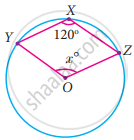Tamil Nadu Board of Secondary EducationSSLC (English Medium) Class 9th

# Find the value of x° in the following figure: - Mathematics

Sum

Find the value of x° in the following figure:#### Solution

Reflex ∠YOZ = 2∠YXZ

= 2(120°)

= 240°

∠YOZ = 360° – reflex ∠YOZ

= 360° – 240°

= 120°

∴ x = 120°

Concept: Theorem: The angle subtended by an arc of the circle at the centre is double the angle subtended by it at any point on the remaining part of the circle.
Is there an error in this question or solution?